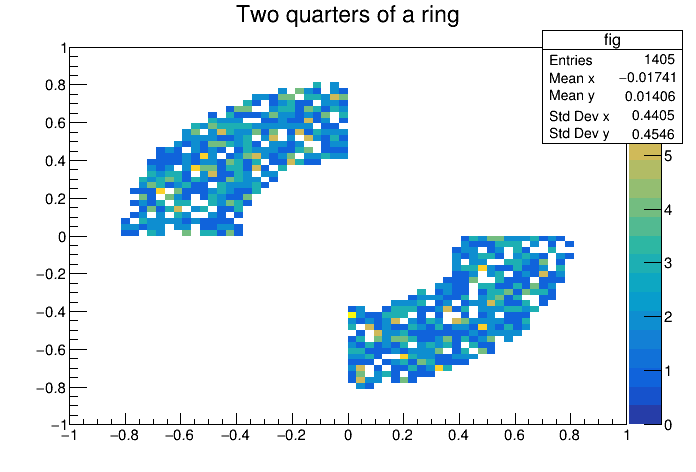# Df 0 1 6_Vec Ops¶

This tutorial shows the potential of the VecOps approach for treating collections stored in datasets, a situation very common in HEP data analysis.

Author: Danilo Piparo
This notebook tutorial was automatically generated with ROOTBOOK-izer from the macro found in the ROOT repository on Monday, July 06, 2020 at 11:36 AM.

In :
import ROOT

df = ROOT.RDataFrame(1024)
coordDefineCode = '''ROOT::VecOps::RVec<double> {0}(len);
std::transform({0}.begin(), {0}.end(), {0}.begin(), [](double){{return gRandom->Uniform(-1.0, 1.0);}});
return {0};'''
d = df.Define("len", "gRandom->Uniform(0, 16)")\
.Define("x", coordDefineCode.format("x"))\
.Define("y", coordDefineCode.format("y"))

Welcome to JupyROOT 6.23/01


Now we have in hands d, a RDataFrame with two columns, x and y, which hold collections of coordinates. The size of these collections vary. Let's now define radii out of x and y. We'll do it treating the collections stored in the columns without looping on the individual elements.

In :
d1 = d.Define("r", "sqrt(x*x + y*y)")


Now we want to plot 2 quarters of a ring with radii .5 and 1 Note how the cuts are performed on RVecs, comparing them with integers and among themselves

In :
ring_h = d1.Define("rInFig", "r > .4 && r < .8 && x*y < 0")\
.Define("yFig", "y[rInFig]")\
.Define("xFig", "x[rInFig]")\
.Histo2D(("fig", "Two quarters of a ring", 64, -1, 1, 64, -1, 1), "xFig", "yFig")

cring = ROOT.TCanvas()
ring_h.Draw("Colz")


Draw all canvases

In :
from ROOT import gROOT
gROOT.GetListOfCanvases().Draw()